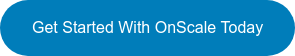# OnScale Blog

Our blog covers tips for using OnScale, new features and developments, and upcoming events and webinars.  Subscribe and get the latest posts in your inbox.

# Theory: How to Calculate Piezoelectric Material Properties from a Material Datasheet

Obtaining the correct piezoelectric material properties from a manufacturer datasheet and transforming those properties into the correct format for simulation can be a hassle.

Let’s take the example of the datasheet below. We will show you how to convert the soft ceramic material "C-6" present on this datasheet into a format which can be inserted into the material database of OnScale.Before we explain how to do that, first we need to look at some of the equations defining piezoelectricity to understand more closely what those material coefficients mean.

## What is the Piezoelectric Effect?

There’s no shame in refreshing our memory before going into the equations, as you will see, it will be extremely useful. The piezoelectric effect is a complex way to say that a pressure on a special material will generate some electric voltage. The piezoelectric effect is a reversible process so if you apply a voltage at the 2 extremities of a piezo material, it will then deform mechanically.## Equations of Piezoelectricity

The piezoelectric effect that we previously described can be translated into 2 important behavior laws which describe the combined effect of electrical and elastic mechanical behavior.

The linear electrical behavior of the material:• D is the electric charge density displacement (electric displacement)
• ε is permittivity (free-body dielectric constant)
• E is electric field strength

The Hooke’s law for elastic materials:• S is strain
• s is compliance under short-circuit conditions
• T is stress

These relations may be combined into so-called coupled equations, of which the strain-charge form is:Of course, we need to think about those equations in terms of their matrix equivalent.

## Strain-charge Matrix relation for the tetragonal PZT C4V

For example, the strain-charge for a material of the 4mm (C4v) crystal class (such as a poled piezoelectric ceramic such as tetragonal PZT or BaTiO3) as well as the 6mm crystal class may also be written as (ANSI IEEE 176):These are the relations we will use to practically calculate the coefficients afterwards, so those matrices are very important.

## The 4 Constitutive Piezoelectric equations

In practice, piezoelectric coefficients can be defined in four ways as follows:And because of that, we can define the previous 2 equations in 4 different "formats" in function of the coefficients we want to use:Following is a description of all matrix variables used in the piezoelectric constitutive equations:

 Symbol Object Type Size Units Meaningvector 6 x 1stress components (e.g. s1)vector 6 x 1strain components (e.g. e3)vector 3 x 1electric field componentsvector 3 x 1electric charge density displacement componentsmatrix 6 x 6compliance coefficientsmatrix 6 x 6stiffness coefficientsmatrix 3 x 3electric permittivitymatrix 3 x 6piezoelectric coupling coefficients for Strain-Charge form

What to do with all that?

In a second, more practical approach, Part 2 will show you:

1- Which coefficients OnScale need

2- A practical algorithm in Python to calculate all those coefficients

3- How to input them in OnScale as a new custom piezoelectric material

Ready for Part 2 of this blog tutorial? We start by answering what parameters are needed in OnScale to define a Piezoelectric material. Click here to start reading!###### Cyprien Rusu, Director of Engineering for Asia at OnScale
Cyprien Rusu is our Director of Engineering for Asia at OnScale. He has a extensive background in FEM, Technical Marketing, Sales and Support. Cyprien recieved his MS in Civil Engineering from Tsinghua University. At OnScale he is a trusted advisor for our client base in Asia, while creating and providing OnScale training.